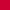This article originally appeared in the January 2002 issue of GammOnLine. Thank you to Kit Woolsey for his kind permission to reproduce it here.

## Sample Size and Computer Roll-Outs

By DeWayne Derryberry, Ph.D
Mathematics and Computer Science
University of Puget Sound

### Introduction

Every backgammon game has (ignoring the doubling cube), one of six outcomes: a player may win a backgammon, gammon, or single game (+3, +2, +1) or lose a backgammon, gammon or single game (-3, -2, -1). The true equity of any move is the average of the outcomes of every possible game.

Computer roll-outs such as those available with Jellyfish and Snowie (bots), often used to determine the equity of a move or position, are not as precise as some people may assume. In fact, there are limits to what a roll-out can accomplish. In a roll-out, a computer plays a position many times starting with each of two alternative moves. The move that receives the highest equity in the roll-out is deemed the better move.

In any one game there is no equity, only a specific outcome – a win or loss of 1,2, or 3 points. If we perform a roll-out, and average the outcomes, we get a sample equity, which is an approximation of the actual equity associated with a move. But the sample equity, based on many outcomes, is not exactly the true equity, based on all possible outcomes.

There are two elements at work in the calculation of sample equity – the underlying equity of each move (signal) and chance variation (noise). As we use roll-outs of greater length, true equity – the average of all possible outcomes – begins to dominate chance variation. In thinking properly about roll-outs it is critical to distinguish between sample equity (which varies from roll-out to roll-out) and true equity (which never changes).

### Sample Size and Equity

If one move is much better than another, a roll-out based on only a few games will show this, it two moves are close in a true equity, even very long roll –outs, based on many complete games, may have trouble yielding a conclusion. If this is intuitively obvious, you should become a statistician, you have a good intuition for the randomness around us. Most non-statisticians are unaware of this key point. No specific length of roll-out can always determine a best move.

An analogous situation occurs all the time in the news. In political opinion polls the same sample size is always used, but this fixed sample size can only predict the winner in one-sided elections. For example, if a poll were to show Bush with 48% of the vote and Gore with 45%, with a margin of error of 3% (a common margin of error indicating a sample size of about 1,000 to 2,000) that means Bush will get 45% to 51% of the vote and Gore 42% to 48% of the vote. Too close to call! On the other hand, if Bush were to get 60% and Gore 35%, even with the 3% margin of error, we know Bush will win, we expect him to get at least 57% and Gore at most 38%. Bush will win by a wide margin.

To predict a winner under the first scenario, Bush favored by 48% of the sample of voters and Gore favored by 45%, we would need a much smaller margin of error, and hence a much larger sample of voters.

Roll-outs are a sampling process as well, subject to chance variation, and only accurate to a margin of error that shrinks with increased sample size, but never goes away. But where is this “margin of error” in roll-outs?

### What's the Best Move?

We can include the margin of error in our comparisons of different moves using a process called a statistical hypothesis test. We assume two moves are really equal (have the same true equity), and only declare one move better, if the difference (in sample equity) we see is statistically significant – the difference is more likely due to a real difference in the true equity of the moves themselves and not just chance variation. (The details of this test are included in the appendix, the main body assumes no knowledge of statistics.)

A relationship between sample size and difference in sample equity has the following form:

n = the number of games played in the roll-out
d = the difference in equity for two moves, based on the roll-out (sample equity).

For minimal evidence one move is better than another we need: n(d)2 > 15, and for overwhelming evidence we need: n(d)2 > 30.

### Examples

Suppose I generate a roll-out involving 20,000 complete games for each of two moves and find move #1 has sample equity 0.041 and move #2 has sample equity 0.034. Calculating we find: 20,000(0.041 - 0.034)2 = 0.98, which is much less than 15. I do not have much evidence at all that move #1 is better than move #2! Any apparent differences (in sample equity) may well be due to chance variation. In other words, someone else, using another software, a different random number generator, or different settings would get slightly different results and might very well find move #2 better.

Another way of saying this: although the sample equity is different for the two moves, the difference is not so great that we can say the true equities differ, or which move really has the higher true equity.

Suppose, on the other hand, I roll out a position 2,000 times and find move #1 has sample equity 0.20 and move #2 has sample equity 0.68. Calculating, we find:

2,000(0.20 - 0.68)2 = 460, which is much greater than 30. In this case, although the roll-out is based on a small number of games, the evidence in overwhelming. Move #2 is clearly better. Someone else using a different software, different random number generator, or different settings, will (almost certainly) reach the same conclusion. Results may vary slightly, but move #2 will always be clearly better.

Another way of saying this: the differences in sample equity are so great that, although we do not know the true equities exactly, we know that move #2 has a much higher true equity.

Notice that a small sample is sufficient to find the best move when the moves have vastly different equity, but a large sample is NOT enough when the moves are close in equity.

### What is the True Equity of a Move?

Can we estimate the true equity of a move, based on the sample equity? We can, using a confidence interval. A confidence interval uses the sample equity plus or minus a margin of error to estimate the true equity.

For any roll-out result we can estimate the true equity by (see appendix):

sample equity ± 3/Ön.

For example, in the first case above we had a roll-out involving 20,000 games and the first move had sample equity 0.041 and the second move had sample equity of 0.034. The estimated true equities are

Move #1: 0.041 ± 3/141.4 = 0.020 to 0.062
Move #2: 0.034 ± 3/141.4 = 0.013 to 0.055.

The considerable overlap between the two intervals is additional evidence that the two moves are indistinguishable at this sample size. Although, move #1 appears to have higher equity, the equity for move #1 could be as low as 0.020 and the equity for move #2 could be as high as 0.055.

### Absolute Limitations

Roll-outs can only detect the better move when the combined values of n (the number games completed in the roll-out) and d (the difference in sample equity between the two moves) reach a certain threshold. If “d” is small, we may never get an “n” big enough. Bots have pseudo-random number generators, and pseudo-random number generators have a period. After a certain point, the dice rolls just repeat and no new scenarios/outcomes are generated. If you perform roll-out more times than the period of the random number generator, you are just repeating old scenarios, not creating any new ones.

In other words, all bots have a largest n, and this implies that for very small differences, n(d)2 can never exceed 15. So moves with nearly identical sample equities cannot be compared using bot roll-outs.

### Summary

All sampling schemes, including computer roll-outs, have a common statistical issue. Are apparent differences due primarily to chance variation, or a true difference, indicative of long-run behavior? I have presented some simple rules for determining when a roll-out gives enough information that we can say one move is better than another – when we can rule out, for the most part, that one move just appears better due to chance variation.

I give a rule of thumb as follows:

n = the number of games played in the roll-out
d = the difference in sample equity for the two moves.

If n(d)2 > 15 there is some evidence the move that appears better really is better.
If n(d)2 > 30, there is overwhelming evidence the move that appears better really is better.

I also have a rule of thumb for estimating the true equity of a move from the sample equity (roll-out results).

n = the number of games played in the roll-out
e = the equity based on the roll-out (sample equity),

e ±3/Ön.

For statistics fanatics, the mathematical derivation of these rules can be found in the Appendix.

### Appendix

The hypothesis test:

The following ideas are based on independent two-sample t-tests, with very large sample sizes (so that we can use a z-table in place of a t-table). Denote the following: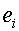--the true equity for move i. The average of all outcomes if we rolled-out the position an “infinite number of times”.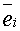--the sample equity for move i, found in the roll-out. The average of the outcomes in the roll-out.

We are considering a statistical hypothesis test where the null and alternative hypotheses are:

Ho: ei = ej versus  Ha: ei ą ej.

The test statistic is, which should be approximately normal (see comments at the end).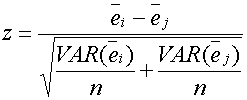The variance for each move can be estimated by assuming some typical outcomes.

Assume a player either wins or loses a backgammon 1% of the time, either wins or loses a gammon 13% of the time, and either wins or loses a single game 36% of the time. In this case the variance in equity outcomes is 1.94 points. Although each position and move has a different profile of outcomes, most positions have a similar variance.

The value z = 1.96 is associated with a p-value (significance level) of 5%. If there is really no difference in the true equity of the two moves we will see this large an apparent difference, due to chance variation, 5% of the time. This is widely acknowledged to be the point (if we must pick a set point) where we claim we have evidence something more than chance variation is going on.

The value z = 2.58 is associated with a p-value (significance level) of 1%. If there is really no difference in the true equity of the two moves we will see this large an apparent difference, due to chance variation, a mere 1% of the time. This is widely acknowledged to be the point (if we must pick a set point) where we claim we have strong evidence something more than chance variation is going on.

Putting this all together, we have evidence when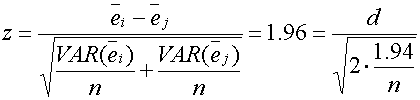In other words n(d)2 = 14.9 is the borderline case, for statistical significance at the 5% level. I simplify this to n(d)2 = 15.

For the second case, we have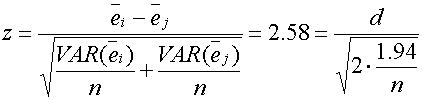In other words n(d)2 = 25.8 is the borderline case, for statistical significance at the 1% level. I simplify this to n(d)2 = 30, which is actually associated with a p-value of 0.54%, and makes a nicer rule of thumb, especially since 30 is twice 15.  This I consider overwhelming (very strong?) evidence.

The confidence interval:

The most common confidence interval is 95%. Indicating that such confidence intervals, in the long run, tend to be wrong 5% of the time.  A 95% confidence interval, assuming a normal distribution (see below) for any average (for example, the sample equity), is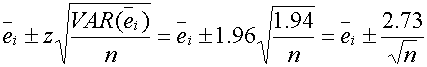The rule is easier to remember if 2.73 is replaced with 3, which is actually a 97% confidence interval.

The normality assumption:  Because the outcomes of a game are actually a profile of 6 possible outcomes (-3, -2, -1 for losses and +1, +2, + 3 for wins), and because the number of outcomes in a roll-out is usually in the thousands, we can say the test statistic is normally distributed.